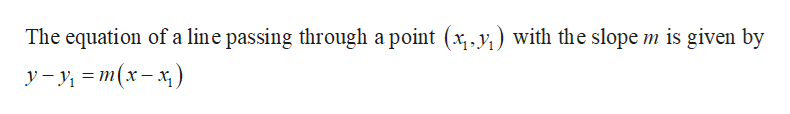# Find the equation of the line though the point (3,3) and perpendicular to the line y=52x−10. Write your answer in the form y=mx+b.

Question
1 views

Find the equation of the line though the point (3,3) and perpendicular to the line y=52x−10. Write your answer in the form y=mx+b.

check_circle

Step 1

Slope of the line y = 52x – 10 is 52 and so the slope of a lin...help_outlineImage TranscriptioncloseThe equation of a line passing through a point (x y) with the slope m is given by y=y = m(x-x) fullscreen

### Want to see the full answer?

See Solution

#### Want to see this answer and more?

Solutions are written by subject experts who are available 24/7. Questions are typically answered within 1 hour.*

See Solution
*Response times may vary by subject and question.
Tagged in

### Other